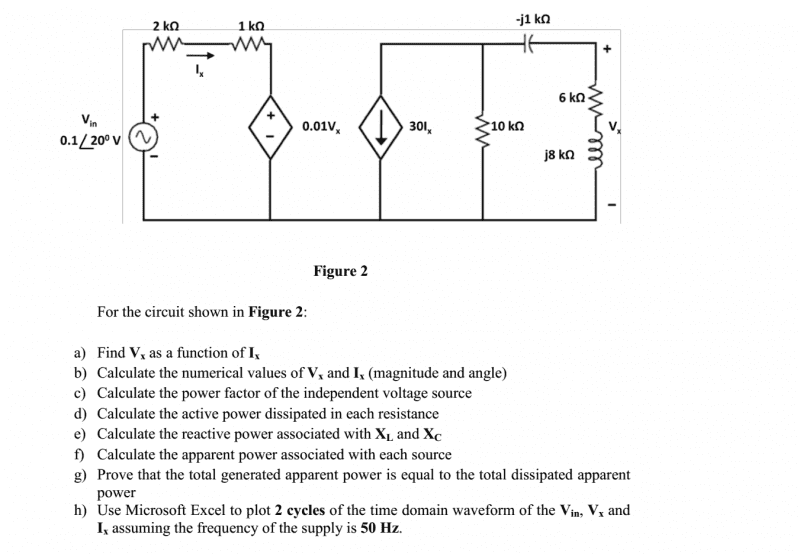# AC Circuit with Dependent Voltage and Current Sources

• Engineering
blackhawk93
Warning! Posting template must be used for homework questions, some work must be shown.
I have trouble solving the following problem. Am I supposed to start by doing nodal analysis on the loop on the right. Can someone give me a start. Thanks....## Answers and Replies

For part (a) you can apply KVL to loop 1 and thus can get a relation between Vx and Ix.

•blackhawk93
For second part hint is that you need to calculate current across series impedance of resistor and inductor, by doing so you can calculate Vx in terrms of Ix. Now you have two relations of Vx and Ix , solve simultaneous equations and get the values of Vx and Ix.

•blackhawk93
blackhawk93
Thank y
For second part hint is that you need to calculate current across series impedance of resistor and inductor, by doing so you can calculate Vx in terrms of Ix. Now you have two relations of Vx and Ix , solve simultaneous equations and get the values of Vx and Ix.
For part (a) you can apply KVL to loop 1 and thus can get a relation between Vx and Ix.
Thank you so much.. I did the first part now moving on to the 2nd part.

blackhawk93
Cant figure out part (g)